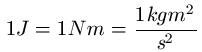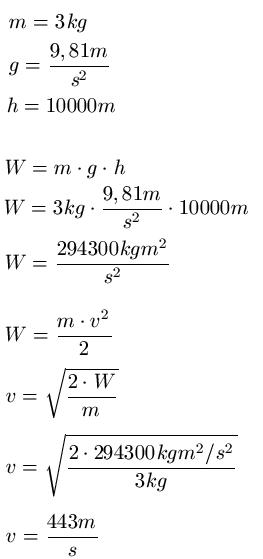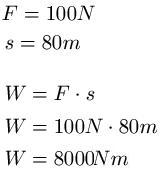# How is potential energy measured

## Potential and Kinetic Energy (Physics)

In this section we deal with potential energy and kinetic energy. In this context we also deal with the concept of work.Everyone knows the term "work" from their everyday lives. You do your job, for many people that equates to eight hours sitting in an office and doing your own job. There is also a job in physics. However, this refers to how much energy it takes to push or lift an object. The work is in joules, newton meters or even in kgm2: s2 specified. The three statements express the same thing, when calculating only the use of one or the other spelling can be of interest. Again to write with:In the following I try to explain the kinetic and potential energy as simply as possible. If you have problems understanding it, I advise you to read the following articles again. These contain knowledge that should be helpful in understanding energy:

Potential and kinetic energy:

Show:

### Potential energy, kinetic energy

Let's come to potential energy. This is understood to be the energy that you have to apply to lift an object a certain height. Example: I lift the television 1 meter up to place it on the table. How much work do I have to put into this? The answer is the formula for potential energy.

Calculate potential energy:

• W.POT = m * g * h
• "WPOT"is the potential energy in Newton meters [Nm]
• "m" is the mass of the body being lifted in kilograms [kg]
• "g" is the acceleration due to gravity, g = 9.81m / s2 [m / s2 ]
• "h" is the height by which the object is lifted in meters [m]

If you put mass, acceleration due to gravity and altitude into the formula, you get the potential energy. If you drop the object, it gets faster and faster (because the acceleration of gravity accelerates the object). With the kinetic energy you can now calculate the speed that the object has when it hits the ground. Example: You lift a television set by 1 meter and - accidentally of course - drop it down. You have been able to calculate the potential energy with the formula from above. You use this energy in the formula for the kinetic energy and convert this formula according to the velocity v in order to calculate the impact velocity.

Calculate kinetic energy:

• W.KIN = 0.5 m v2
• W.KIN is the kinetic energy in Newton meters [Nm]
• "m" is the mass of the object in kilograms [kg]
• "v" is the speed in meters per second [m / s]

Example:
A 3 kg block falls from an airplane that flies 10 km above the ground. At what speed does the block hit the ground if you neglect the air resistance?

Solution: We use the formulas for potential and kinetic energy to calculate the speed. This calculation looks like this:Show:

### Work, strength and distance

Work is not only done when lifting an object. Even if you push an object on the floor, you have to do work. There is a formula that combines work, strength and distance.

Calculate work on a route:

• W = F s
• "W" is the work to be done in Newton meters [Nm]
• "F" is the force in Newtons [N]
• "s" is the distance that the object is pushed, in meters [m]

Example:

A body is pushed a distance of 80 meters with a force of 100 N. How much work was done?

Solution: We take all the information for the calculation from the text and insert them into the associated formula:Left:

### Who's Online

We have 571 guests online